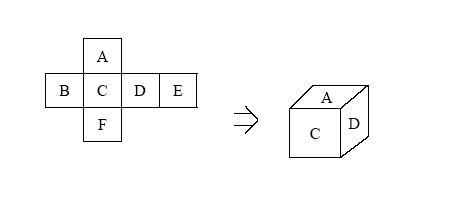Online JudgeProblem SetAuthorsOnline ContestsUser
Web Board
F.A.Qs
Statistical Charts
Problems
Submit Problem
Online Status
Prob.ID:
Register
Authors ranklist
Current Contest
Past Contests
Scheduled Contests
Award Contest
Register
Language:
Dice Stacking
 Time Limit: 1000MS Memory Limit: 65536K Total Submissions: 1594 Accepted: 391

Description

Small John is playing a dice stacking game. Six faces of a die are squares of the same size; each face of a die has a number range from 1 to 6.The dice game is to stack several dice up with arbitrary order. You must keep the following rule: For each pair of consecutive dice, the number on the bottom of the top die must match the number on the top of the bottom die. In other words, the two faces that are together must have the same number.

Then a long rectangular pillar, which has 4 sides, is created. We are trying to make one side have the maximum sum. (Note that after we rotate a die to fix the top face and bottom face, we can still rotate the die by 90, 180, or 270 degrees.) Write a program to find the maximum sum that one side can have.

Input

The first line of the input contains a single integer t, the number of test cases, followed by the input data for each test case. The first line for each test case contains an integer n (1 <= n <= 10), the number of dice. In the next lines, each line contains six integers for a die, by the order A, B, C, D, E, F, as in the following figure. There is a single space between two numbers.

Output

There should be one line per test case, which contains the maximum sum of the numbers in one side. If you cannot stack all the dice up, simply output 0 in a line.

Sample Input

```1
5
2 3 1 6 5 4
3 1 2 4 6 5
5 6 4 1 3 2
1 3 6 2 4 5
4 1 6 5 2 2
```

Sample Output

`30`

Source

[Submit]   [Go Back]   [Status]   [Discuss]Home PageGo BackTo top